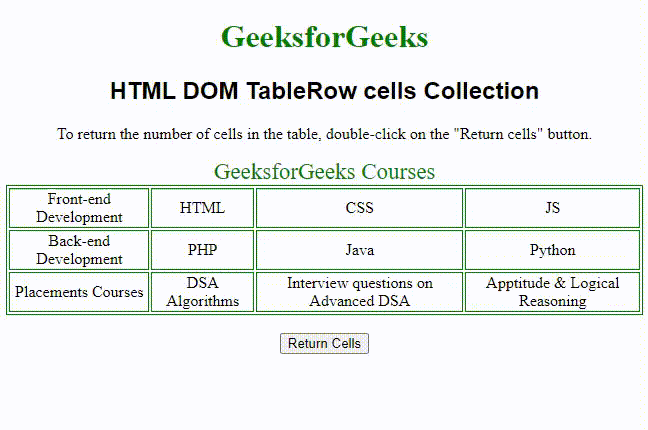Open in App
Not now

# HTML DOM TableRow cells Collection

• Last Updated : 20 Nov, 2021

The HTML DOM TableRow cells Collections is used to return all the total number of cells of a particular row in the table. It includes all the <td> and <th> element. The sequence of the <tr> elements is sorted in the same way as their position in the source code.

Syntax:

`tableObject.cells`

Properties:

• length: It is used to return the number of <td> and <th> element in the collection.

Methods:

• [index]: It is used for returning the <td> and <th> element from the collection with a specified index. If the index number is out of range then it will return null.
• item(index): It is also used for returning the <td> and <th> element from the collection with a specified index. If the index number is out of range then it will return null.
• namedItem(id): It is also used for returning the <td> and <th> element from the collection with a specified id. If the id doesn’t exist then the return value is null.

Return value:- It is used to return all the <td> and <th> element of a particular row in the table.

The below example illustrates the implementation of the TableRow cells collection.

Example:

## HTML

 ```<``html``>``  ``<``head``>``    ``<``title``>TableRow cells Collection in HTML``    ``<``style``>``      ``table,``      ``td {``        ``border: 1px solid green;``      ``}``      ``h1 {``        ``color: green;``      ``}``      ``h2 {``        ``font-family: sans-serif;``      ``}``      ``body {``        ``text-align: center;``      ``}``    ````  ``` `  ``<``body``>``    ``<``h1``>GeeksforGeeks``    ``<``h2``>HTML DOM TableRow cells Collection``    `  `<``p``>``      ``To return the number of cells in the table,``      ``double-click on the "Return cells" button.``    ```   `    ``<``table` `id``=``"Courses"` `align``=``"center"``>``      ``<``caption``>``        ``GeeksforGeeks Courses``      ````      ``<``tr``>``        ``<``td``>Front-end Development``        ``<``td``>HTML``        ``<``td``>CSS``        ``<``td``>JS``      ````      ``<``tr``>``        ``<``td``>Back-end Development``        ``<``td``>PHP``        ``<``td``>Java``        ``<``td``>Python``      ````      ``<``tr``>``        ``<``td``>Placements Courses``        ``<``td``>DSA Algorithms``        ``<``td``>Interview questions on Advanced DSA``        ``<``td``>Aptitude & Logical Reasoning``      ````    ````    ``<``br` `/>` `    ``<``button` `onclick``=``"rowCount()"``>Return Cells` `    ``<``p` `id``=``"test"``>`    `    ``<``script``>``      ``function rowCount() {``        ` `        ``// Number of rows.``        ``var row = document.getElementById("Courses")``            ``.rows.cells.length;``        ``document.getElementById("test").innerHTML =``          ``" Number of cells in the 3rd row: " + row;``      ``}``    ````  `````

Output:Supported Browsers:

• Google Chrome 1.0 & above
• Internet Explorer 5.5 & above
• Microsoft Edges 12.0 & above
• Firefox 1.0 & above
• Safari 3.0 & above
• Opera 12.1 & above

My Personal Notes arrow_drop_up